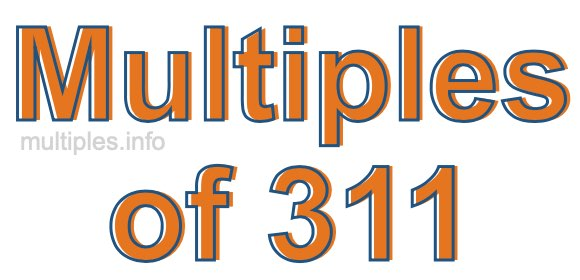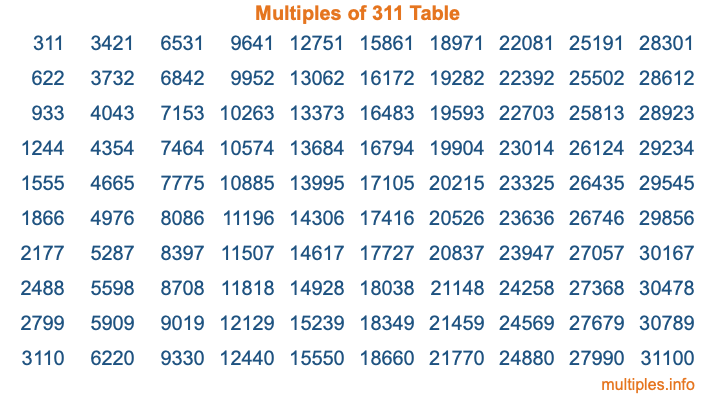Multiples of 311Welcome to the Multiples of 311 page. Here we will first teach you everything you will ever need to know about the multiples of 311, and then give you a study guide summary of everything we taught you to make sure you remember it all. Use this page to look up facts and learn information about the multiples of 311. This page will make you a multiples of three hundred eleven expert!

Definition of Multiples of 311
Multiples of 311 are all the numbers that when divided by 311 equal an integer. Each of the multiples of 311 are called a multiple. A multiple of 311 is created by multiplying 311 by an integer.

Therefore, to create a list of multiples of 311, you start with 1 multiplied by 311, then 2 multiplied by 311, then 3 multiplied by 311, and so on for as long as you want. Thus, the list of the first five multiples of 311 is 311, 622, 933, 1244, and 1555. To see a larger list of multiples of 311, see the printable image of Multiples of 311 further down on this page. We also have a category where you can choose any nth multiple of 311.

Multiples of 311 Checker
The Multiples of 311 Checker below checks to see if any number of your choice is a multiple of 311. In other words, it checks to see if there is any number (integer) that when multiplied by 311 will equal your number. To do that, we divide your number by 311. If the the quotient is an integer, then your number is a multiple of 311.

Is  a multiple of 311?

Least Common Multiple of 311 and ...
A Least Common Multiple (LCM) is the lowest multiple that two or more numbers have in common. This is also called the smallest common multiple or lowest common multiple and is useful to know when you are adding our subtracting fractions. Enter one or more numbers below (311 is already entered) to find the LCM.

Check out our LCM Calculator if you need more details about the Least Common Multiple or if you need the LCM for different numbers for adding and subtraction fractions.

nth Multiple of 311
As we stated above, 311 is the first multiple of 311, 622 is the second multiple of 311, 933 is the third multiple of 311, and so on. Enter a number below to find the nth multiple of 311.

th multiple of 311

Multiples of 311 vs Factors of 311
311 is a multiple of 311 and a factor of 311, but that is where the similarities end. All postive multiples of 311 are 311 or greater than 311. All positive factors of 311 are 311 or less than 311.

Below is the beginning list of multiples of 311 and the factors of 311 so you can compare:

Multiples of 311: 311, 622, 933, 1244, 1555, etc.

Factors of 311: 1, 311

As you can see, the multiples of 311 are all the numbers that you can divide by 311 to get a whole number. The factors of 311, on the other hand, are all the whole numbers that you can multiply by another whole number to get 311.

It's also interesting to note that if a number (x) is a factor of 311, then 311 will also be a multiple of that number (x).

Multiples of 311 vs Divisors of 311
The divisors of 311 are all the integers that 311 can be divided by evenly. Below is a list of the divisors of 311.

Divisors of 311: 1, 311

The interesting thing to note here is that if you take any multiple of 311 and divide it by a divisor of 311, you will see that the quotient is an integer.

Multiples of 311 Table
Below is an image of the first 100 multiples of 311 in a table. The table is in chronological order, column by column. The first column has the first ten multiples of 311, the second column has the next ten multiples of 311, and so on.The Multiples of 311 Table is also referred to as the 311 Times Table or Times Table of 311. You are welcome to print out our table for your studies.

Negative Multiples of 311
Although not often discussed or needed in math, it is worth mentioning that you can make a list of negative multiples of 311 by multiplying 311 by -1, then by -2, then by -3, and so on, to get the following list of negative multiples of 311:

-311, -622, -933, -1244, -1555, etc.

Multiples of 311 Summary
Below is a summary of important Multiples of 311 facts that we have discussed on this page. To retain the knowledge on this page, we recommend that you read through the summary and explain to yourself or a study partner why they hold true.

There are an infinite number of multiples of 311.

A multiple of 311 divided by 311 will equal a whole number.

311 divided by a factor of 311 equals a divisor of 311.

The nth multiple of 311 is n times 311.

The largest factor of 311 is equal to the first positive multiple of 311.

311 is a multiple of every factor of 311.

311 is a multiple of 311.

A multiple of 311 divided by a divisor of 311 equals an integer.

311 divided by a divisor of 311 equals a factor of 311.

Any integer times 311 will equal a multiple of 311.

Multiples of a Number
Here you can get the multiples of another number, all with the same attention to detail as we did for multiples of 311 on this page.

Multiples of
Multiples of 312
Did you find our page about multiples of three hundred eleven educational? Do you want more knowledge? Check out the multiples of the next number on our list!